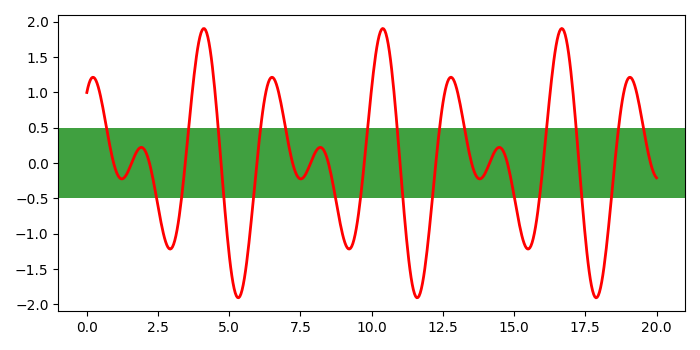# Shading an area between two points in a Matplotlib plot

To shade an area between two points in matplotlib, we can take the following steps−

• Create x and y data points using numpy.
• Plot x and y data points, with color=red and linewidth=2.
• To shade an area parallel to X-axis, initialize two variables, y1 and y2.
• To add horizontal span across the axes, use axhspan() method with y1, y2, green as shade color,and alpha for transprency of the shade.
• To display the figure, use show() method.

## Example

import numpy as np
import matplotlib.pyplot as plt
plt.rcParams["figure.figsize"] = [7.00, 3.50]
plt.rcParams["figure.autolayout"] = True
x = np.linspace(0, 20, 500)
y = np.cos(3*x) + np.sin(2*x)
plt.plot(x, y, c='red', lw=2)
y1 = 0.5
y2 = -0.5
plt.axhspan(y1, y2, color='green', alpha=0.75, lw=0)
plt.show()

## OutputUpdated on: 06-May-2021

4K+ Views